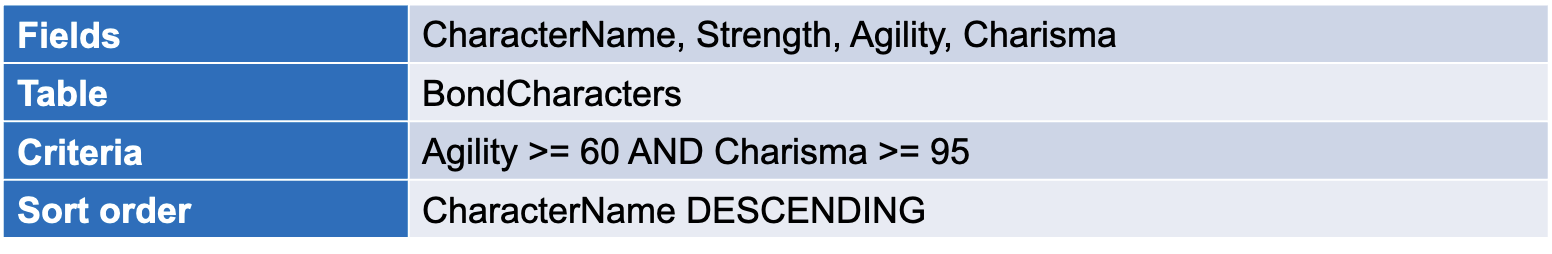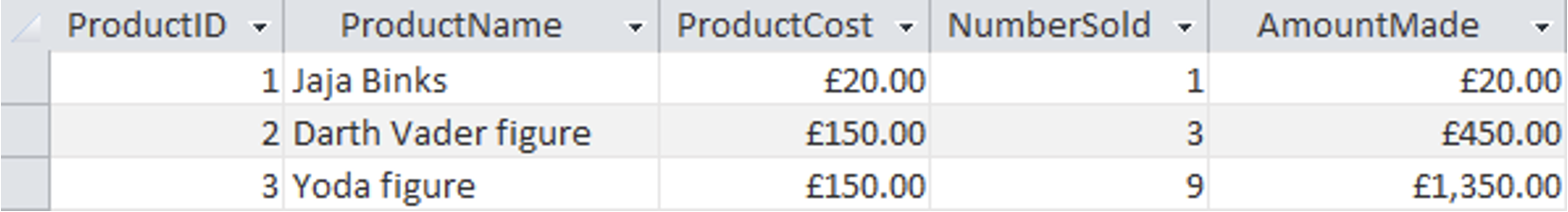# Calculated Fields

## Searching and sorting a database

1. Find everyone who has 60 or more agility and 95 or more charisma and display their name, strength, agility and charisma from the BondCharacters table sorted by their names in reverse order (Z to A).

## Searching and sorting a database

1. Find everyone who has 60 or more agility and 95 or more charisma and display their name, strength, agility and charisma from the BondCharacters table sorted by their names in reverse order (Z to A).## Learning Intentions

• Understand what a calculated field is
• Understand the benefits of calculated fields

## Success Criteria

• I can create a database which could include a calculated field
• I can create a database calculated field

## Parking lot database

• The below table is like something used for a parking lot company which monitors the number of spaces in each parking lots daily. Rather than simply storing the number of spaces available, they calculate the number of spaces available. They do this using a calculated field that calculates the number left using:
TotalSpaces - NumberOfSpacesInUse

## Parking lot database

• The previous table is like something used for a parking lot company which monitors the number of spaces in each parking lots daily. Rather than simply storing the number of spaces available, they calculate the number of spaces available. They do this using a calculated field that calculates the number left using:
TotalSpaces - NumberOfSpacesInUse
Which field is the calculated field below?
How is the calculated field calculated?

Which field is the calculated field below?

How is the calculated field calculated?
NumberSold * ProductCost## Why bother with calculated fields?

• Saves us having to update the database
• They lead to fewer errors in calculations
• They can do complex calculations in very little time

## Calculated fields in Microsoft Access

• Did you notice that we had a Calculated option when selecting the field types in Design View?

## Calculated fields in Microsoft Access

``````TotalSpaces - NumberOfSpacesInUse

ProductCost * NumberSold``````
• The following are examples of calculated fields.

## The Stock table

• What’s the Calculated field here?
• How is it calculated?

## The Stock table

• What’s the Calculated field here? Reorder
• How is it calculated? If the QuantityInStock drops below the ReorderLevel field, we get a Yes

## If statements

• IIf(QuantityInStock < ReorderLevel,"Yes","No")

Write an expression to calculate the average stat for our Top Trumps. In this case, you have Strength, Agility and Intelligence fields. Calculate the average of all three of them.
How would you write a calculation to figure out if a car park was full or not?

Write an expression to calculate the average stat for our Top Trumps. In this case, you have Strength, Agility and Intelligence fields. Calculate the average of all three of them.
(Strength + Agility + Intelligence) / 3
How would you write a calculation to figure out if a car park was full or not?
IIf(NumberOfSpacesInUse = TotalSpaces, "Full", "Spaces available“)

Copy across the parking database file to your own area.
Open the database and complete the tasks.

Presentation Overview
S3 Computer Science :: Databases :: Calculated fields